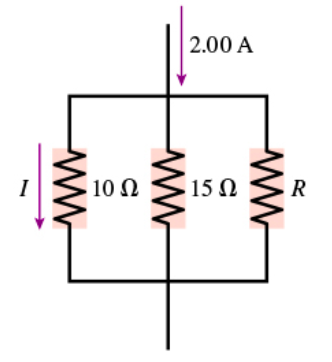# Problem: Part A Consider the circuit shown in (Figure 1). Assume I = 0.96 A What is the value of resistor R?

###### FREE Expert Solution

Ohm's law:

$\overline{){\mathbf{V}}{\mathbf{=}}{\mathbf{i}}{\mathbf{R}}}$

The voltage across the 10Ω resistor is:

V10 = (0.96)(10) = 9.6 V

The resistors are connected in parallel. The potential difference across them is 9.6 V.

93% (365 ratings)###### Problem Details

Part A Consider the circuit shown in (Figure 1). Assume I = 0.96 AWhat is the value of resistor R?